##### Geometry: 1,001 Practice Problems For Dummies (+ Free Online Practice)To find the orthocenter of a triangle, you need to find the point where the three altitudes of the triangle intersect. In the following practice questions, you apply the point-slope and altitude formulas to do so.

## Practice questions

Use your knowledge of the orthocenter of a triangle to solve the following problems.

1. The coordinates of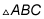are A (0, 2), B (–2, 6), and C (4, 0). Find the coordinates of the orthocenter of this triangle.

2. The coordinates of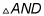are A (0, 0), N (6, 0), and D (–2, 8). Find the coordinates ofthe orthocenter of this triangle.

1. (–8, –6)

The orthocenter of a triangle is the point where the three altitudes of the triangle intersect. An altitude of a triangle is perpendicular to the opposite side. Because perpendicular lines have negative reciprocal slopes, you need to know the slope of the opposite side. Here's the slope of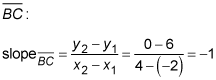This means that the slope of the altitude to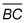needs to be 1.

The point-slope formula of a line is y y1 = m (x x1), where m is the slope and (x1, y1) are the coordinates of a point on the line. To find the altitude formed when you connect Point A to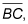plug in m = –1 and the coordinates of Point A, (0, 2):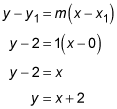The slope of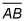is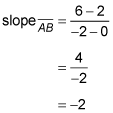This means that the slope of the altitude to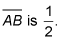The altitude formed when you connect Point C, (4, 0), tois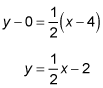To find the orthocenter, you need to find where these two altitudes intersect. Set them equal and solve for x: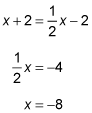Now plug the x value into one of the altitude formulas and solve for y: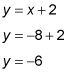Therefore, the altitudes cross at (–8, –6).

2. (–2, –2)

The orthocenter of a triangle is the point where the three altitudes of the triangle intersect. An altitude of a triangle is perpendicular to the opposite side. Because perpendicular lines have negative reciprocal slopes, you need to know the slope of the opposite side. Here's the slope of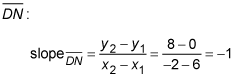This means that the slope of the altitude to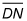needs to be 1.

The point-slope formula of a line is y y1 = m (x x1), where m is the slope and (x1, y1) are the coordinates of a point on the line. To find the altitude formed when you connect Point A to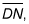plug in m = 1 and the coordinates of A, (0, 0):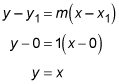Now find the equation for the altitude to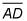The slope of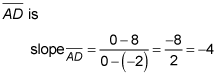This means that the slope of the altitude to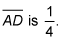The altitude formed when you connect Point N, (6, 0), to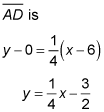To find the orthocenter, you need to find where the two altitudes intersect. Set them equal and solve for x: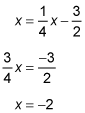Now plug the x value into one of the altitude formulas and solve for y:

y = x

y = –2

Therefore, the altitudes cross at (–2, –2).